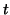Next: Models Up: tr01cb1 Previous: tr01cb1

# Introduction

Functional magnetic resonance imaging studies are typically used to address questions about activation effects in populations of subjects. This generally involves a multi-subject and/or multi-session approach where data are analysed in such a way as to allow for hypothesis tests at the group level [9,19], e.g. in order to assess whether the observed effects are common and stable across or between groups of interest.

Calculating the level and probability of brain activation for a single subject is typically achieved using a linear model of the signal together with a Gaussian noise model for the residuals. This model is commonly referred to as the General Linear Model (GLM) and much attention to date has been focussed on ways of modelling and fitting the (time-series) signal and residual noise at the individual single-session level [2,17,18].

In order to carry out higher-level anlayses it is straightforward to formulate a complete single-level GLM that relates various parameters of interest at the group-level to the full set of (time-series) data available [3,13]. In FMRI, where the human and computational costs involved in data analysis are relatively high, however, it is desirable to be able to make group-level inferences using the results of separate first-level analyses without the need to re-analyse any of the individual subject data. Within such a two-level approach, group parameters of interest can then easily be refined as more data become available. The natural question to address is if and how a two-level approach to group analysis restricts the type of hypotheses we are able to infer at the group level.

In order to be able to generate results that extend to the population, we also need to account for the fact that the individual subjects themselves are sampled from the population and thus are random quantities with associated variances. It is exactly this step that marks the transition from a simple fixed-effects model to a mixed-effects model1 and it is imperative to formulate a model at the group level that allows for the explicit modelling of these additional variance terms [9,5].

In this paper we revisit the single-level mixed-effect GLM for FMRI group analysis which relates the effect of interest at the group level (e.g. difference of patients and controls) to the individual FMRI time-series. This model is well known and encompasses both the familiar single-subject models as well as a general group model [9,3,4,13]. By using the full GLM at the group level, one obtains a very general and flexible framework which allows us to examine more complex relationships beyond the simple tests such as mean group activation. An example of a more general group analysis would be to test whether activation is correlated with some other variable such as drug dosage or disability score.

The main result shows that this single-level GLM can be decomposed into an equivalent two-level version so that group analyses can be performed using only the lower-level parameter estimates and their (co-)variances, from the individual subject analyses. This result is formally stated in terms of a model equivalence theorem. The practical consequence is that it is possible to perform valid group analyses in two stages: firstly the individual subject analyses, and secondly, a single group analysis performed on the output of the combined estimates from the individual subject analyses. Furthermore, there are very few restrictions placed upon the model and so it can be used in very general conditions, such as when the estimates at the individual subject level were obtained using different pre-whitening for each subject or indeed different regressors. We finally give examples of how, in this general framework, group tests of interest, like paired or unpaired-tests, can easily be formulated. The model equivalence result applies not only to FMRI studies but to any mixed-effects GLM that can be split into two levels in the same way as presented here.

All models used here are instances of the univariate GLM. This means treating each single, registered voxel separately and assuming Gaussian noise. Parameter estimation is being performed (at all levels) using pre-whitening approach [2,17] which is known to be the best linear unbiased estimator (BLUE) for these models .

In this paper we make a distinction between the problems involved in modelling of FMRI data at the group level and estimation of the relevant (co-)variances. While estimation of variances is an important issue when implementing such models, we consider the problems involved as beyond the scope of this paper. A companion paper on practical issues of estimation, using Bayesian methods, is currently being prepared and these techniques form the basis of what has been implemented in the latest software release of FSL .Next: Models Up: tr01cb1 Previous: tr01cb1
Christian Beckmann 2003-07-16